Courses

# NCERT Solutions Chapter 2 - Theory of Consumer Behaviour, Class 12, Economics | EduRev Notes

## Commerce : NCERT Solutions Chapter 2 - Theory of Consumer Behaviour, Class 12, Economics | EduRev Notes

The document NCERT Solutions Chapter 2 - Theory of Consumer Behaviour, Class 12, Economics | EduRev Notes is a part of the Commerce Course Economics Class 12.
All you need of Commerce at this link: Commerce

Question 1: What do you mean by the budget set of a consumer?
It refers to the set of consumption bundles that are available to or affordable by the consumer; while being aware of his/her income-level and the existing market prices.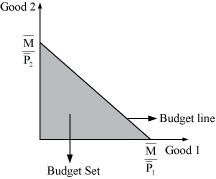Question 2: What is a budget line?
A budget line represents the different combinations of two goods that are affordable and are available to a consumer; while being aware of his/her income-level and market prices of both the goods.
Let x1 be the amount of good 1.
x2 be the amount of good 2.
P1 be the price of good 1.
P2 be the price of good 2.
P1x1 = Total money spent on good 1
P1x2 = Total money spent on good 2
Then, the budget line will be:
P1x1 + P2x2 = M
All the consumption bundles on the budget line cost the consumer exactly the equivalent of hi/her income.

Question 3 : Explain why the budget line is downward sloping.
The budget line is a negatively downward sloping line. The slope of a budget line measures the amount of good 2 that must be sacrificed in order to get an additional unit of good 1, as the consumer’s income (M) is fixed. The budget line is downward sloping because, in order to increase the consumption of one good, the consumption of the other good must be reduced, with constant M.
The slope of the budget line is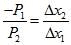, which implies the rate of exchange or the rate at which good 2 can be substituted for good 1.

Question 4 : A consumer wants to consume two goods. The prices of the two goods are Rs 4 and Rs 5 respectively. The consumer’s income is Rs 20.
(i) Write down the equation of the budget line.
(ii) How much of good 1 can the consumer consume if he/she spends his/her entire income on that good?
(iii) How much of good 2 can be consumed if he/she spends his/her entire income on that good?
(iv) What is the slope of the budget line?

Answer : (i) P1 = Rs 4
P2 = Rs 5
M = Rs 20
Equation of the budget line = P1x1 + P2x2 = M
4x1 + 5x2=20
(ii) If Rs 20 is entirely spent on good 1, then the amount of good 2 demanded will be zero i.e., x2 = 0 as the consumer has no income left to spend on good 2.
4x1 + 5 (0) = 20
4x1 = 20

x1 = 20/4

x1 = 5
Amount of good 1 consumed = 5 units
(iii) If Rs 20 is entirely spent on good 2, then x1 = 0, as the consumer has no income left to spend on good 1.
4(0) + 5(x2) = 20

5(x2) = 20

x2 = 20/5
x2 = 4 units
Amount of good 2 consumed = 4 units
(iv) Slope of the budget line =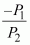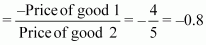Question 5 : How does the budget line change if the consumer’s income increases to Rs 40 but the prices remain unchanged?

M2 = Rs 40
P1 = Rs 4
P2 = Rs 5
Initial equation of the budget line:
4x1 + 5x2 = 20
New equation of the budget line:
4x1 + 5x2 = 40
As M has increased, the consumer can now purchase more of both the goods and the budget line will shift parallelly outwards to A’B’ from AB.
Horizontal intercept will be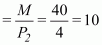Vertical intercept will be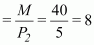The slope of the new budget line will be the same as that of the old budget line.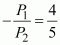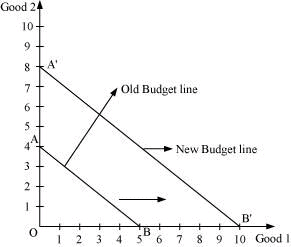Question 6 : How does the budget line change if the price of good 2 decreases by a rupee but the price of good 1 and the consumer’s income remain unchanged?
Answer : P1 = Rs 4
P2 = Rs 5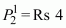M = Rs 20
Since the income and the price of good 1 are unchanged, the decrease in the price of good 2 will increase the vertical intercept of the budget line. The new budget line will also pivot outwards around the same horizontal intercept.
Horizontal intercept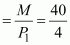Vertical interceptSlope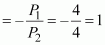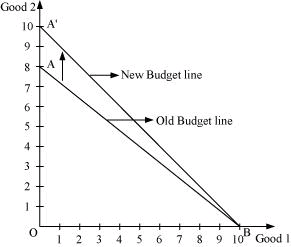The slope of the new budget line will be more and the new budget line will be steeper than the original one.

Question 7 : What happens to the budget set if the prices as well as the income double?
Answer : If the prices and the income are doubled, then the budget line will remain unchanged.

 M1 = Rs 20 M2 = Rs 40 P1 = Rs 4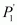= Rs 8 P2 = Rs 5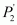= Rs 10
Horizontal intercept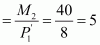Vertical intercept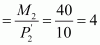Slope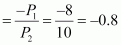Hence, the vertical intercept, the horizontal intercept and the slope of the budget line will remain the same. The new budget line will be the same as the old budget line but associated with higher income and higher prices of both the goods.

Question 8 : Suppose a consumer can afford to buy 6 units of good 1 and 8 units of good 2 if he/she spends her entire income. The prices of two goods are Rs 6 and Rs 8. How much is the consumer’s income?
P1 = Rs 6
P2 = Rs 8
x1 = 6
x2 = 8
Budget line = M = P1x1 + P2x2
M = 6 × 6 + 8 × 8
M = 36 + 64
M = 100
Thus, the consumer’s income is Rs 100.

Question 9 : Suppose a consumer wants to consume two goods that are available only in integer units. The two goods are equally priced at Rs 10 and the consumer’s income is Rs 40.
(i) Write down all the bundles that are available to the consumer.
(ii) Among the bundles that are available to a consumer, identify those that cost will him/her exactly Rs 40.

Answer : (i) P1 = Rs 10
P2 = Rs 10
M = Rs 40
Budget set ⇒ P1x1 + P2x2M
10x1 + 10x2 ≤ 40

The bundles that are available to the consumer should cost less than or equal to Rs 40.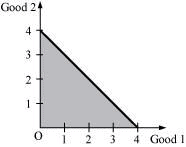Horizontal intercept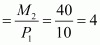Vertical intercept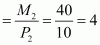Slope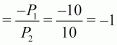The bundles in the shaded region (ΔAOB) are all available to the consumer, including the bundles lying on the line AB.

 (0, 0) (0, 1) (0, 2) (0, 3) (0, 4) (1, 0) (1, 1) (1, 2) (1, 3) (1, 4) (2, 0) (2, 1) (2, 2) (2, 3) (2, 4) (3, 0) (3, 1) (3, 2) (3, 3) (3, 4) (4, 0) (4, 1) (4, 2) (4, 3) (4, 4)
(ii) The coordinates that lie on the line AB cost exactly the same as the income of the consumer. The bundles are as follows:
(0, 4), (1, 3), (2, 2), (3, 1), (4, 0)
Question 10 : What do you mean by monotonic preferences?
It means that the consumer prefers a particular bundle over the other bundle if the former consists of at least more of one good and no less of the other good.
Example:If bundle A(3, 5) and bundle B(3, 2) are available to the consumer, then he/she will prefer bundle A over bundle B as bundle A consists of more units of good 2 than bundle B.
Question 11 : If the consumer has monotonic preferences, then can he/she be indifferent towards bundles (10, 8) and (8, 6)?
No, he/she cannot be indifferent towards these two bundles as bundle I consists of more of both goods as compared to bundle II. He/she will prefer bundle I over bundle II as it contains 10 units of good 1 and 8 units of good 2 as compared to 8 units and 6 units of good 1 and good 2 respectively in bundle II.
Page No : 35
Question 12 : Suppose a consumer’s preferences are monotonic. What can you say about his/her preference ranking over the bundles (10, 10), (10, 9) and (9, 9)?

 Bundles U1 I) (10, 10) 3 II) (10, 9) 2 III) (9, 9) 1

As the consumer’s preferences are monotonic, more is better and he/she will prefer bundle I over the rest of the bundles. This means that bundle I will be assigned a higher utility number i.e., three (rank = three) out of the available three bundles.

Question 13 : Suppose your friend is indifferent to the bundles (5, 6) and (6, 6). Are the preferences of your friend monotonic?
It is given that my friend is indifferent towards the bundles (5, 6) and (6, 6). This implies that his/her preferences are not monotonic. If he/she is indifferent towards both the bundles, then it means that he/she derives the same level of satisfaction and assigns them the same rank. However, the second bundle consists of more of both the goods. Thus, according to the monotonicity assumption, he/she must prefer the second bundle over the first.

Question 14 : Suppose there are two consumers in the market for a good and their demand functions are as follows:d1(p) = 20 − p for any price less than or equal to 20 and d1(p) = 0 at any price greater than 20.
d2(p) = 30 − 2p for any price less than or equal to 15 and d1(p) = 0 at any price greater than 15.
Find out the market demand function.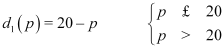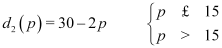For price less than Rs 15 (p 15)
Market demand for a good = d1 (p) + d2 (p)
= 20 − p + 30 − 2p
= 50 − 3p
For price more than Rs 15 but less than Rs 20 (15 <p 20)
Market demand = d1(p) + d2 (p)
= 20 − p + 0    (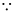for p > 15, d2 (p) = 0)
= 20 − p
For price more than Rs 20 (p > 20)
Market demand = d1(p) + d2 (p)
= 0 + 0    (for p > 10, d1 (p) = 0, d2 (p) = 0)
= 0
Thus, market demand
= 50 − 3p if p ≤ 15
=20 − p if 15 < p ≤ 20
= 0 if p > 20
Question 15 : Suppose there are 20 consumers for a good and they have identical demand functions:
D(p) = 10 − 3p for any price less than or equal to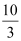and d1(p) = 0 at any price greater than.
What is the market demand function?
d(p) = 10 − 3p if pd1 (p) = 0 if p >Market demand = Summation of demand of all the consumers in the market

For priceMarket demand = 20 ∑d (p)    (Since consumers have identical demand curve)

= 20 × (10 − 3p)
= 200 − 60p
For price >Market demand = 20 × d1(p)
= 20 × 0

= 0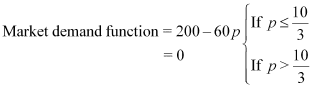Question 16 : Consider a market where there are just two consumers and suppose their demands for the good are given as follows:
Calculate the market demand for the goods.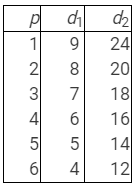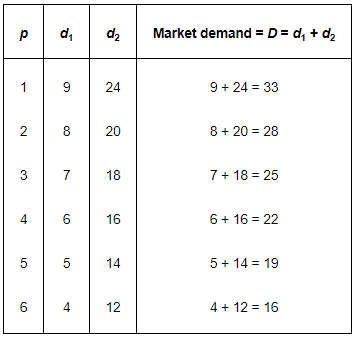Question 17 : What do you mean by a normal good?
Answer : Those goods that share a positive relationship with income but a negative relationship with price are called normal goods. In other words, if the income of a consumer increases, then the demand for a normal good also increases. However, the demand will fall with the rise in the price of that good.
That is,
If the price of a good (Px) increases, thenthedemand for good (Dx) decreases
If a consumer’s income (M) increases, then thedemand for good Dx increases.

Question 18 : What do you mean by an ‘inferior good’? Give some examples.
Inferior good: Those goods that share an inverse relationship with their prices and with the income of a consumer are called inferior goods. That is,
If the price of a good (Px) increases, then thedemand for good (Dx) decreases.
If a consumer’s income (M) increases, then the demand for good (Dx) decreases.
Examples: Coarse cereals, bidis etc.

Question 19 : What do you mean by substitutes? Give examples of two goods which are complements of each other.
Those goods that can be consumed in place of other goods are called substitute goods. Example: Tea and coffee are goods that can be substitutes for each other. If the price of tea increases, then the demand for tea will decrease and people will substitute coffee for tea, which will increase the demand for coffee.
The demand for a good moves in the same direction as the price of its substitutes.
Price of tea (PT) increases Demand for tea (DT) decreases Demand for coffee (DC) increases.

Question 20: What do you mean by complements? Give examples of two goods which are complements of each other.
Those goods that are consumed together are called complementary goods. Example: Tea and sugar. If the price of sugar increases, then it will lead to a decrease in the demand for tea. If the price of tea increases, then it will reduce the demand for sugar. The demand for a good moves in the opposite direction of the price of its complementary goods. That is, If the price of tea (PT) increases, then the demand for sugar (DS) decreases. If the price of sugar (PS) increases, then the demand for tea (DT) decreases.

Question 21 : Explain price elasticity of demand.
Price elasticity of demand is the measure of the degree of responsiveness of the demand for a good to the changes in its price. It is defined as the percentage change in the demand for a good divided by the percentage change in its price.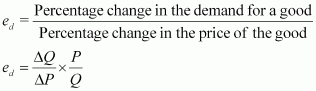Where,

ΔQ = Q2Q1, change in demand

ΔP = P2 P1, change in demand

P = Initial price

Q = Initial quantity

Question 22 : Consider the demand for a good. At price Rs 4, the demand for the good is 25 units. Suppose the price of the good increases to Rs 5, and as a result, the demand for the good falls to 20 units. Calculate the price elasticity.

 P1 = 4P2 = 5ΔP = P2 − P1= 5 − 4= 1 Q1 = 25Q2 = 20ΔQ = Q2 − Q1= 20 − 25= −5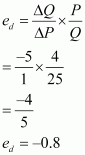Question 23 : Consider the demand curve D(p) = 10 − 3p. What is the elasticity at price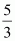?
D(p) = 10 − 3p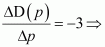Change in demand per unit change in price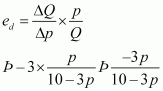At price p =,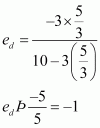i.e., the elasticity of demand at price p =is unitary elastic.

Question 24 : Suppose the price elasticity of demand for a good is −0.2. If there is a 5% increase in the price of the good, then by what percentage will the demand for the good go down?
ed = −0.2    [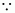Note that ed = −2. Hence, we need not prefix ed to (−2)]
Percentage change in price = 5%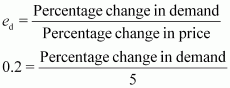1.0 ⇒ Percentage change in demand
= 1%

Question 25 : Suppose the price elasticity of demand for a good is −0.2. How will the expenditure on the good be affected if there is a 10% increase in its price?
Answer : Price elasticity of demand = −0.2
Percentage increase in price = 10%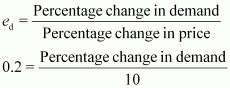−2 = Percentage change in demand
Thus, percentage decrease in demand is less than the percentage increase in price. This means that when price increases and ed < 1, the demand is inelastic and hence, the expenditure will increase.

Question 26 : Suppose there was a 4% decrease in the price of a good, and as a result, the expenditure on the good increased by 2%. What can you say about the elasticity of demand?
Percentage decrease in price = 4%
Increase in expenditure = 2%
ΔE= ΔP {q + (1 + ed)}
Since the price has decreased, the expenditure on the good will increase. This implies that the percentage of change in demand has increased more than the percentage decrease in price.
Thus, elasticity =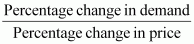The numerator is more than the denominator. This means that elasticity is more than 1. We can say that the small change in price has lead to a bigger change in demand, and as a result, the demand is elastic.

Offer running on EduRev: Apply code STAYHOME200 to get INR 200 off on our premium plan EduRev Infinity!

,

,

,

,

,

,

,

,

,

,

,

,

,

,

,

,

,

,

,

,

,

,

,

,

,

,

,

;